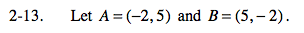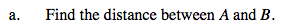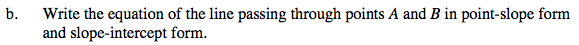### Home > CAAC > Chapter 13 > Lesson 13.PSF1-S > Problem2-13

2-13.Plot the points and connect them. Then create a right triangle with segment AB as the hypotenuse.
Use the Pythagorean Theorem to calculate the length of segment AB.1. Determine the slope: rise/run.
2. Substitute a given point and the slope into the point-slope formula.
3. Solve for y for the slope-intercept form.